HLIBpro  2.3.1
Solving an Integral Equation
Remarks
This example corresponds to the C++ example "Solving an Integral Equation".

In this example, an integral equation is to be solved by representing the discretised operator with an 𝓗-matrix, whereby the 𝓗-matrix is constructed using a matrix coefficient function for entries of the equation system. Furthermore, for the iterative solver a preconditioner is computed using 𝓗-LU factorisation.

# Problem Description

Given the integral equation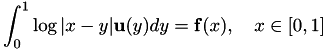with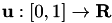being sought for a given right hand side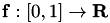, the Galerkin discretisation with constant ansatz functions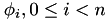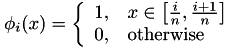leads to a linear equation system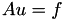where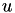contains the coefficients of the discretised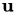and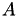is defined byThe right hand side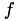is given by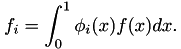Remarks
This example is identical to the introductory example in [BoerGraHac:2003b]}.

# Coordinates and Cluster Trees

The code starts with the standard initialisation:

#include <stdio.h>
#include <hlib-c.h>
int main ( int argc, char ** argv )
{
int info;
hlib_init( & info ); CHECK_INFO;

Together with the initialisation, the verbosity level of HLIBpro was increased to have additional output during execution. The macro CHECK_INFO is defined as

#define CHECK_INFO { if ( info != HLIB_NO_ERROR ) \
{ char buf; hlib_error_desc( buf, 1024 ); \
printf( "\n%s\n\n", buf ); exit(1); } }

and tests the result of a 𝓗𝖫𝖨𝖡𝗉𝗋𝗈 function call. If an error occured, the description of this error is queried using hlib_error_desc and printed to the screen.

For clustering the unknowns, coordinate information is to be defined. For this 1d example, the indices are placed in the middle of equally sized intervals.

int i;
int n = ... /* set problem dimension */
double h = 1.0 / ((double) n);
double ** vertices;
hlib_coord_t coord;
vertices = (double**) malloc( n * sizeof(double*) );
for ( i = 0; i < n; i++ )
{
vertices[i] = (double*) malloc( sizeof(double) );
vertices[i] = h * ((double) i) + (h / 2.0);
}
coord = hlib_coord_import( n, 1, vertices, NULL, & info ); CHECK_INFO;

Coordinates in HLIBpro are vectors of the corresponding dimension, e.g. one in for this problem.

Having coordinates for each index, the cluster tree and block cluster tree can be constructed.

hlib_clustertree_t ct;
hlib_blockclustertree_t bct;
ct = hlib_clt_build_bsp( coord, HLIB_BSP_AUTO, 20, & info ); CHECK_INFO;
bct = hlib_bct_build( ct, ct, adm, & info ); CHECK_INFO;

For the cluster tree, the partitioning strategy for the indices is determined automatically by choosing HLIB_BSP_AUTO. For the block cluster tree, the standard admissibility condition (see {eqn:std_adm}) is used as defined by HLIB_ADM_STD_MIN with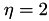.

# Matrix Construction and Coefficient Function

Using the block cluster tree, finally the 𝓗-matrix can be constructed. For this, adaptive cross approximation (ACA, see ???) is applied to compute low rank approximations of admissibly matrix blocks. In HLIBpro, an improved version of ACA, ACA+ is implemented.

ACA only needs access to the matrix coefficients of the dense matrix. Those are provided by a coefficient function kernel with an optional parameter h, which will be discussed below. Furthermore, the block wise accuracy of the 𝓗-matrix approximation has to be defined. In this case, an accuracy of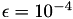is used and stored in acc. Finally, a flag indicates, whether the matrix is symmetric or not.

hlib_matrix_t A;
acc, 1, & info ); CHECK_INFO;

The coefficient function used during matrix construction comes in the form of a callback function:

void
kernel ( const size_t n, const int * rowidx,
const size_t m, const int * colidx,
hlib_real_t * matrix, void * arg )
{
int rowi, colj;
double h = *((double*) arg);
for ( colj = 0; colj < m; colj++ )
{
const int idx1 = colidx[colj];
for ( rowi = 0; rowi < n; rowi++ )
{
const int idx0 = rowidx[rowi];
double value;
if ( idx0 == idx1 )
value = -1.5*h*h + h*h*log(h);
else
{
const double dist = h * ( fabs( (double) (idx0-idx1) ) - 1.0 );
const double t1 = dist+1.0*h;
const double t2 = dist+2.0*h;
value = ( - 1.5*h*h + 0.5*t2*t2*log(t2) - t1*t1*log(t1) );
if ( fabs(dist) > 1e-8 )
value += 0.5*dist*dist*log(dist);
}
matrix[(colj*n) + rowi] = -value;
}
}
}

It is called, whenever matrix coefficients are needed. Usually, a complete block of coefficients is requested by the internal matrix constructor, e.g. a full dense block in case of an inadmissible leaf or a full row/column in case of ACA. The row (column) indices for the requested coefficients are stored in rowidx (colidx) and are of size n (m). In the array matrix the coefficients have to be stored in column-wise order, e.g. the coefficient with the i'th row index and the j'th column index at position (i,j) or at matrix[j*n+i]. The final argument arg is the additional parameter to hlib_matrix_build_coeff at can be used to provided necessary data for the coefficient function. Here, it is the stepwidth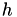of the problem.

For a complex coefficient functions the callback function is identical except the value type of matrix, which is of type hlib_complex_t.

# H-LU Factorisation

Having constructed the 𝓗-matrix, the corresponding equation system shall be solved. In most cases, a standard iterative solve will not be sufficient, e.g. either the convergence rate is to bad or there is no convergence at all. Therefore, a (good) preconditioner is needed, with the inverse ofbeing the best possible. Since iterative schemes, only need matrix-vector multiplication, the corresponding functionality for the inverse is sufficient. This is provided by an (𝓗-) LU factorisation of, or for the symmetric case, a LDL factorisation:

hlib_matrix_t B;
hlib_linearoperator_t A_inv;
B = hlib_matrix_copy( A, & info ); CHECK_INFO;
A_inv = hlib_matrix_factorise_inv( B, acc, & info ); CHECK_INFO;

Since A is modified during the factorisation, a copy B is constructed. For the factorisation the accuracy is chosen to be equal as during matrix construction. The result of the factorisation is linear operator represented by the type hlib_linearoperator_t, which can be used to perform matrix vector multiplication. In this case, the evaluation of the inverse ofis provided.

Remarks
A matrix can always be used as a linear operator, but not the other way around, i.e. a linear operator is not a full matrix object.

# Iterative Solvers

Still missing is the right hand side of the equation system. In this example, it was chosen, such that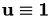:

hlib_vector_t b = hlib_vector_build( n, & info ); CHECK_INFO;
double * b_arr = (hlib_real_t *) malloc( n * sizeof(hlib_real_t) );
for ( i = 0; i < n; i++ )
b_arr[i] = rhs( i, n );
hlib_vector_import( b, b_arr, & info ); CHECK_INFO;
hlib_vector_permute( b, hlib_clt_perm_e2i( ct, & info ), & info ); CHECK_INFO;

Here, hlib_vector_import copies the coefficients from b_arr to b and rhs is defined by

double
rhs ( int i, int n )
{
const double a = ((double)i) / ((double) n);
const double b = ((double)i+1.0) / ((double) n);
double value = -1.5 * (b - a);
if ( fabs( b ) > 1e-8 ) value += 0.5*b*b*log(b);
if ( fabs( a ) > 1e-8 ) value -= 0.5*a*a*log(a);
if ( fabs( 1.0 - b ) > 1e-8 ) value -= 0.5*(1.0-b)*(1.0-b)*log(1.0-b);
if ( fabs( 1.0 - a ) > 1e-8 ) value += 0.5*(1.0-a)*(1.0-a)*log(1.0-a);
return value;
}
Remarks
Instead of using a special array b_arr, the coefficients of b could directly be set using hlib_vector_entry_set.

For the iterative solver the special auto solver is used, which automatically chooses a suitable solver for the given combination of matrix and preconditioner. Furthermore, statistics about the solution process is stored in solve_info, e.g. convergence rate, number of steps.

hlib_vector_t x = hlib_vector_build( n, & info ); CHECK_INFO;
hlib_solver_t solver;
hlib_solve_info_t solve_info;
solver = hlib_solver_auto( & info ); CHECK_INFO;
hlib_solver_solve( solver, (hlib_linearoperator_t) A, x, b,
A_inv, & solve_info, & info ); CHECK_INFO;
Remarks
Solvers in 𝓗𝖫𝖨𝖡𝗉𝗋𝗈 are based only on matrix vector multiplication and hence, only need the functionality of linear operators. This is an example, where matrices can simply be casted to the hlib_linearoperator_t type.

To check the accuracy of the computed solution, we compare it with the known exact solution:

hlib_vector_t one = hlib_vector_build( n, & info ); CHECK_INFO;
double error;
hlib_vector_permute( x, hlib_clt_perm_i2e( ct, & info ), & info ); CHECK_INFO;
hlib_vector_fill( one, 1.0, & info ); CHECK_INFO;
hlib_vector_axpy( 1.0, one, x, & info ); CHECK_INFO;
error = hlib_vector_norm_inf( x, & info ); CHECK_INFO;
printf( " error of solution = %.4e\n", error );

Finally, all resources have to be freed and 𝓗𝖫𝖨𝖡𝗉𝗋𝗈 be finished:

hlib_vector_free( one, & info ); CHECK_INFO;
hlib_vector_free( x, & info ); CHECK_INFO;
hlib_solver_free( solver, & info ); CHECK_INFO;
hlib_vector_free( b, & info ); CHECK_INFO;
hlib_linearoperator_free( A_inv, & info ); CHECK_INFO;
hlib_matrix_free( B, & info ); CHECK_INFO;
hlib_matrix_free( A, & info ); CHECK_INFO;
hlib_bct_free( bct, & info ); CHECK_INFO;
hlib_clt_free( ct, & info ); CHECK_INFO;
hlib_coord_free( coord, & info ); CHECK_INFO;
free( b_arr );
for ( i = 0; i < n; i++ )
free( vertices[i] );
free( vertices );
hlib_done( & info ); CHECK_INFO;
return 0;
}
Remarks
Please note, that the linear operator A_inv and the copy B of A are explicitly freed. By default, A_inv will only use the provided matrix object and does not perform memory management, e.g. deletion.

# The Plain Program

#include <stdio.h>
#include <hlib-c.h>
#define CHECK_INFO { if ( info != HLIB_NO_ERROR ) \
{ char buf; hlib_error_desc( buf, 1024 ); \
printf( "\n%s\n\n", buf ); exit(1); } }
void
kernel ( const size_t n, const int * rowidx,
const size_t m, const int * colidx,
hlib_real_t * matrix, void * arg )
{
int rowi, colj;
double h = *((double*) arg);
for ( colj = 0; colj < m; colj++ )
{
const int idx1 = colidx[colj];
for ( rowi = 0; rowi < n; rowi++ )
{
const int idx0 = rowidx[rowi];
double value;
if ( idx0 == idx1 )
value = -1.5*h*h + h*h*log(h);
else
{
const double dist = h * ( fabs( (double) (idx0-idx1) ) - 1.0 );
const double t1 = dist+1.0*h;
const double t2 = dist+2.0*h;
value = ( - 1.5*h*h + 0.5*t2*t2*log(t2) - t1*t1*log(t1) );
if ( fabs(dist) > 1e-8 )
value += 0.5*dist*dist*log(dist);
}
matrix[(colj*n) + rowi] = -value;
}
}
}
double
rhs ( int i, int n )
{
const double a = ((double)i) / ((double) n);
const double b = ((double)i+1.0) / ((double) n);
double value = -1.5 * (b - a);
if ( fabs( b ) > 1e-8 ) value += 0.5*b*b*log(b);
if ( fabs( a ) > 1e-8 ) value -= 0.5*a*a*log(a);
if ( fabs( 1.0 - b ) > 1e-8 ) value -= 0.5*(1.0-b)*(1.0-b)*log(1.0-b);
if ( fabs( 1.0 - a ) > 1e-8 ) value += 0.5*(1.0-a)*(1.0-a)*log(1.0-a);
return value;
}
int
main ( int argc, char ** argv )
{
int info;
int i;
int n = ... /* set problem dimension */
double h = 1.0 / ((double) n);
double ** vertices;
hlib_coord_t coord;
hlib_clustertree_t ct;
hlib_blockclustertree_t bct;
hlib_matrix_t A, B;
hlib_linearoperator_t A_inv;
hlib_vector_t b;
double * b_arr = NULL;
hlib_vector_t x;
hlib_solver_t solver;
hlib_solve_info_t solve_info;
hlib_vector_t one;
double error;
hlib_init( & info ); CHECK_INFO;
vertices = (double**) malloc( n * sizeof(double*) );
for ( i = 0; i < n; i++ )
{
vertices[i] = (double*) malloc( sizeof(double) );
vertices[i] = h * ((double) i) + (h / 2.0);
}
coord = hlib_coord_import( n, 1, vertices, NULL, & info ); CHECK_INFO;
ct = hlib_clt_build_bsp( coord, HLIB_BSP_AUTO, 20, & info ); CHECK_INFO;
bct = hlib_bct_build( ct, ct, adm, & info ); CHECK_INFO;
acc = hlib_acc_fixed_eps( 1e-4 );
acc, 1, & info ); CHECK_INFO;
B = hlib_matrix_copy( A, & info ); CHECK_INFO;
A_inv = hlib_matrix_factorise_inv( B, acc, & info ); CHECK_INFO;
b = hlib_vector_build( n, & info ); CHECK_INFO;
b_arr = (hlib_real_t *) malloc( n * sizeof(hlib_real_t) );
for ( i = 0; i < n; i++ )
b_arr[i] = rhs( i, n );
hlib_vector_import( b, b_arr, & info ); CHECK_INFO;
hlib_vector_permute( b, hlib_clt_perm_e2i( ct, & info ), & info ); CHECK_INFO;
x = hlib_vector_build( n, & info ); CHECK_INFO;
solver = hlib_solver_auto( & info ); CHECK_INFO;
hlib_solver_solve( solver, (hlib_linearoperator_t) A, x, b,
A_inv, & solve_info, & info ); CHECK_INFO;
hlib_vector_permute( x, hlib_clt_perm_i2e( ct, & info ), & info ); CHECK_INFO;
one = hlib_vector_build( n, & info ); CHECK_INFO;
hlib_vector_fill( one, 1.0, & info ); CHECK_INFO;
hlib_vector_axpy( 1.0, one, x, & info ); CHECK_INFO;
error = hlib_vector_norm_inf( x, & info ); CHECK_INFO;
printf( " error of solution = %.4e\n", error );
hlib_vector_free( one, & info ); CHECK_INFO;
hlib_vector_free( x, & info ); CHECK_INFO;
hlib_solver_free( solver, & info ); CHECK_INFO;
hlib_vector_free( b, & info ); CHECK_INFO;
hlib_linearoperator_free( A_inv, & info ); CHECK_INFO;
hlib_matrix_free( B, & info ); CHECK_INFO;
hlib_matrix_free( A, & info ); CHECK_INFO;
hlib_bct_free( bct, & info ); CHECK_INFO;